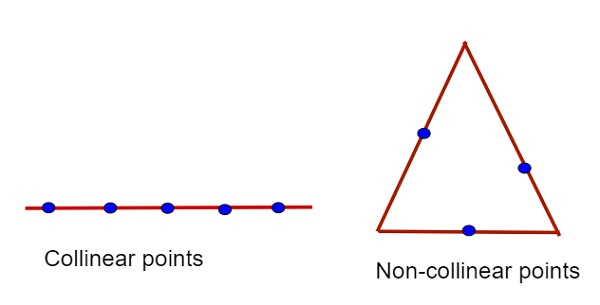# Program to check if three points are collinear in C++

Given with three different valued points and the task is to check whether the points are collinear or not.

Points are said to be collinear if they lie on the same line and they are not collinear if they are on the different lines. Given below is the figure of collinear and non-collinear points.Input

x1 = 1, x2 = 2, x3 = 3, y1 = 1, y2 = 4, y3 = 5

Output

no points are not collinear

Input

x1 = 1, y1 = 1, x2 = 1, y2 = 4, x3 = 1, y3 = 5

Output

points are collinear

## Approach used in the below program is as follow

• Input the points as (x1, y1), (x2, y2), (x3, y3)

• Apply the formula of area of triangle x1 * (y2 - y3) + x2 * (y3 - y1) + x3 * (y1 - y2)

• check for the conditions as −

• if the area of triangle is 0 than print points are collinear

• if the area of triangle is not 0 than print points are not collinear

• print the final result

## Algorithm

Start
Step 1→ declare function to check if points are collinear or not
void check_collinear(int x1, int y1, int x2, int y2, int x3, int y3)
declare int a = x1 * (y2 - y3) + x2 * (y3 - y1) + x3 * (y1 - y2)
IF (a == 0)
Print "yes points are collinear"
End
Else
Print "no points are not collinear"
Step 2→ In main()
Declare int x1 = 1, x2 = 2, x3 = 3, y1 = 1, y2 = 4, y3 = 5
Call check_collinear(x1, y1, x2, y2, x3, y3)
Stop

## Example

Live Demo

#include <bits/stdc++.h>
#include <math.h>
#include <stdlib.h>
using namespace std;
//check if points are collinear or not
void check_collinear(int x1, int y1, int x2, int y2, int x3, int y3){
int a = x1 * (y2 - y3) + x2 * (y3 - y1) + x3 * (y1 - y2);
if (a == 0)
cout << "yes points are collinear";
else
cout << "no points are not collinear";
}
int main(){
int x1 = 1, x2 = 2, x3 = 3, y1 = 1, y2 = 4, y3 = 5;
check_collinear(x1, y1, x2, y2, x3, y3);
return 0;
}

## Output

If run the above code it will generate the following output −

no points are not collinear